# Angles and bearings

### Term 2 starting in week 1 :: Estimated time: 2 weeks

• Use cardinal directions and related angles (review)
• Draw and interpret scale diagrams (review)
• Understand and represent bearings
• Make scale drawings using bearings
• Calculate bearings using angles rules
• Solve bearings problems using Pythagoras and trigonometry

For higher-attaining pupils:

• Solve bearings problems using the sine and cosine rules

This page should remember your ticks from one visit to the next for a period of time. It does this by using Local Storage so the information is saved only on the computer you are working on right now.

## Lesson Starters

Here are some suggestions for whole-class, projectable resources which can be used at the beginnings of each lesson in this block.

### 1st Lesson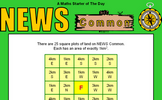#### News Common

Follow the compass directions given in the squares to find where the route starts?

### 2nd Lesson#### Air Traffic Control

Work out which aircraft are in danger of colliding from their positions and direction of travel. An exercise in understanding bearings.

### 3rd Lesson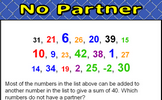#### No Partner

Find which numbers in a given list do not combine with other numbers on the list to make a given sum.

### 4th Lesson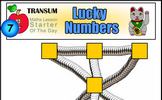#### Pipeline Puzzle

Arrange the numbers so that the totals of the three numbers along any pipe are the same.

### 5th Lesson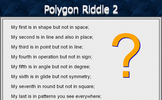#### Polygon Riddle 2

A "My first is in..." type riddle leading to a polygon interior angle calculation.

### 6th Lesson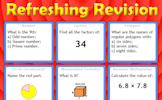#### Refreshing Revision

It is called Refreshing Revision because every time you refresh the page you get different revision questions.

Some of the Starters above are to reinforce concepts learnt, others are to introduce new ideas while others are on unrelated topics designed for retrieval practice or and opportunity to develop problem-solving skills.

White Rose ResourcesEnd of block assessments provide a quick progress check at the end of each block of learning to make sure students have understood the content covered. This Scheme of Learning was produced by White Rose Maths and is used here with permission granted on 30th June 2021.For All: